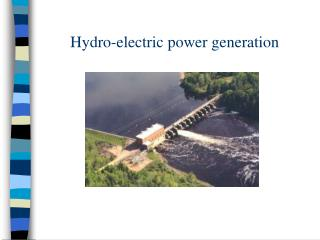Download PresentationHydro-electric power generation

# Hydro-electric power generation - PowerPoint PPT PresentationDownload Presentation## Hydro-electric power generation

- - - - - - - - - - - - - - - - - - - - - - - - - - - E N D - - - - - - - - - - - - - - - - - - - - - - - - - - -
##### Presentation Transcript

1. Hydro-electric power generation

2. Snowy Mountains Scheme. • The Snowy Mountains Scheme consists of 7 power stations - Murray 1, Murray 2, Guthega, Blowering, Tumut 1 (located 366 metres below ground level), Tumut 2 (located 244 metres below ground level), and Tumut 3. • These power stations have 31 turbines with a total generating capacity of 3756 megawatts (MW).

3. Schematic of a Hydro-electric power station.

4. Water flow throw turbines. • The electrical energy that is generated at the dams power plant is a result of the converting the potential energy of the water behind the dam in to electrical energy. Water from behind the dam is allowed to stream through pipes near the bottom of the dam. The pipes connect through turbines so that the water turns the turbines which produces the electricity.

5. Calculating Power • Amount of Energy: GPE = mgh • Definition of Power: It is rate at which energy is transferred. • Density of water is defined as the mass per unit volume.

6. Calculating Power • Assuming the water falls 200m we can use the expressions for power and gravitational potential energy to calculate the power actually generated, given the rate of flow of water through the turbines.

7. Calculating the Rate of flow

8. The Hoover Dam in the USA • The 17 turbines at the Hoover Dam are capable of producing 2000 Megawatts of power. • Estimate the amount of water that flows through the turbines.

9. Calculating the Flow Rate for the Hoover Dam.